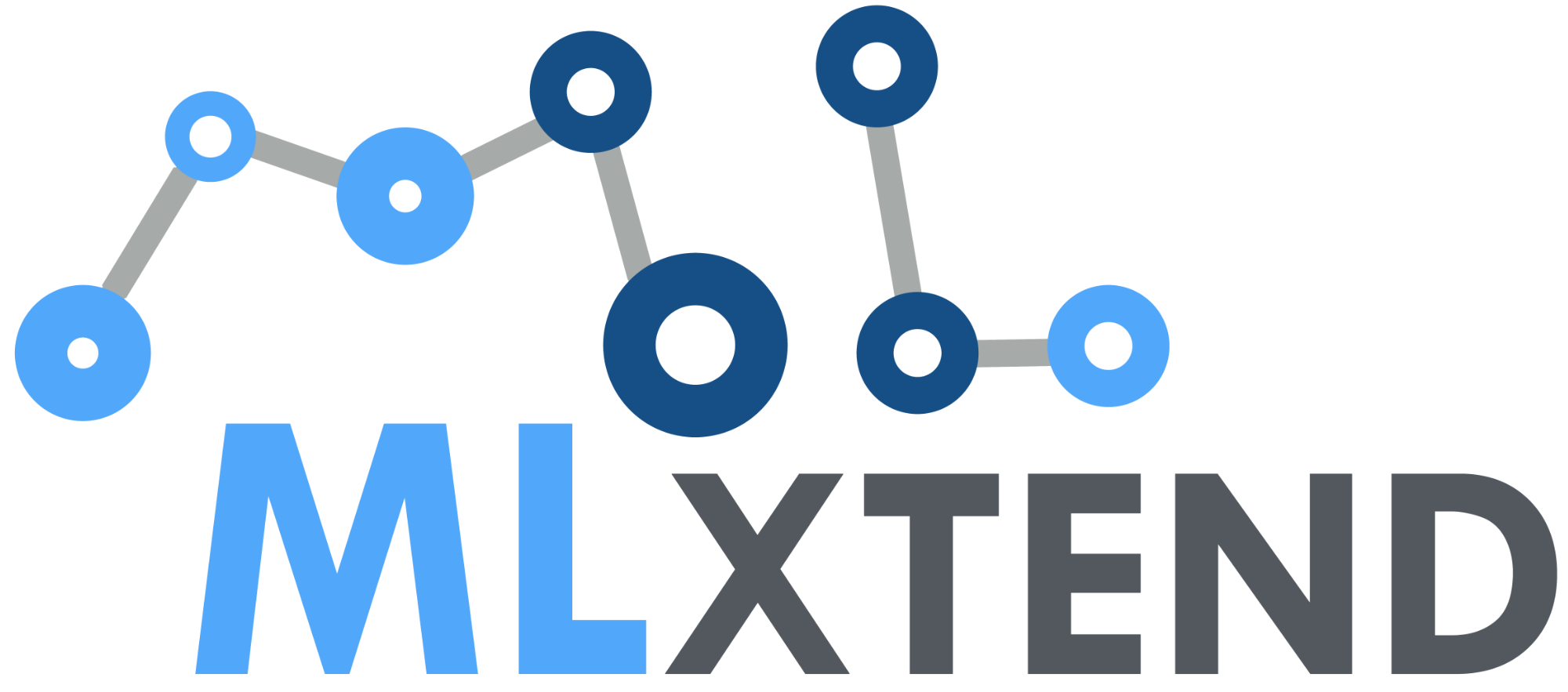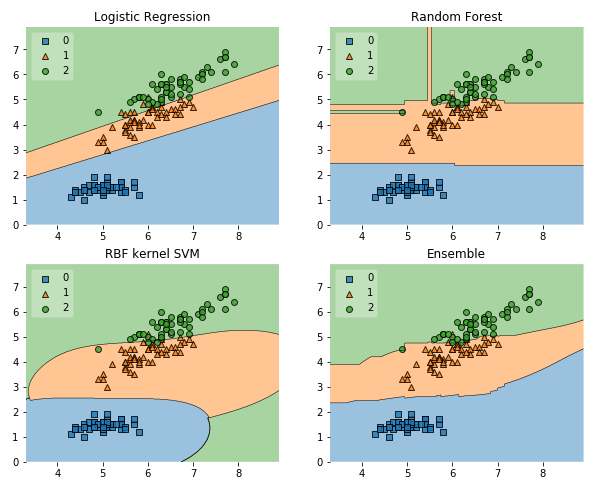### Welcome to mlxtend's documentation!

Mlxtend (machine learning extensions) is a Python library of useful tools for the day-to-day data science tasks.## Examples

import numpy as np
import matplotlib.pyplot as plt
import matplotlib.gridspec as gridspec
import itertools
from sklearn.linear_model import LogisticRegression
from sklearn.svm import SVC
from sklearn.ensemble import RandomForestClassifier
from mlxtend.classifier import EnsembleVoteClassifier
from mlxtend.data import iris_data
from mlxtend.plotting import plot_decision_regions

# Initializing Classifiers
clf1 = LogisticRegression(random_state=0)
clf2 = RandomForestClassifier(random_state=0)
clf3 = SVC(random_state=0, probability=True)
eclf = EnsembleVoteClassifier(clfs=[clf1, clf2, clf3],
weights=[2, 1, 1], voting='soft')

X, y = iris_data()
X = X[:,[0, 2]]

# Plotting Decision Regions

gs = gridspec.GridSpec(2, 2)
fig = plt.figure(figsize=(10, 8))

labels = ['Logistic Regression',
'Random Forest',
'RBF kernel SVM',
'Ensemble']

for clf, lab, grd in zip([clf1, clf2, clf3, eclf],
labels,
itertools.product([0, 1],
repeat=2)):
clf.fit(X, y)
ax = plt.subplot(gs[grd, grd])
fig = plot_decision_regions(X=X, y=y,
clf=clf, legend=2)
plt.title(lab)

plt.show()If you use mlxtend as part of your workflow in a scientific publication, please consider citing the mlxtend repository with the following DOI:@article{raschkas_2018_mlxtend,
author       = {Sebastian Raschka},
title        = {MLxtend: Providing machine learning and data science
utilities and extensions to Python’s
scientific computing stack},
journal      = {The Journal of Open Source Software},
volume       = {3},
number       = {24},
month        = apr,
year         = 2018,
publisher    = {The Open Journal},
doi          = {10.21105/joss.00638},
url          = {http://joss.theoj.org/papers/10.21105/joss.00638}
}